Mathematics MATRICES FOR CBSE - NCERT
Click for Only Video

### Topic covered

star Matrix
star Types of matrix

### Matrix

\color{green} ✍️ A matrix is an ordered rectangular array of numbers or functions.

\color{green} ✍️ The numbers or functions are called the elements or the entries of the matrix
\color{green} ✍️ We denote matrices by capital letters. The following are some examples of matrices:● In the examples, the horizontal lines of elements are said to constitute, rows of the matrix and the vertical lines of elements are said to constitute, columns of the matrix.

●Thus A has 3 rows and 2 columns, B has 3 rows and 3 columns while C has 2 rows and 3 columns.

### Order of a matrix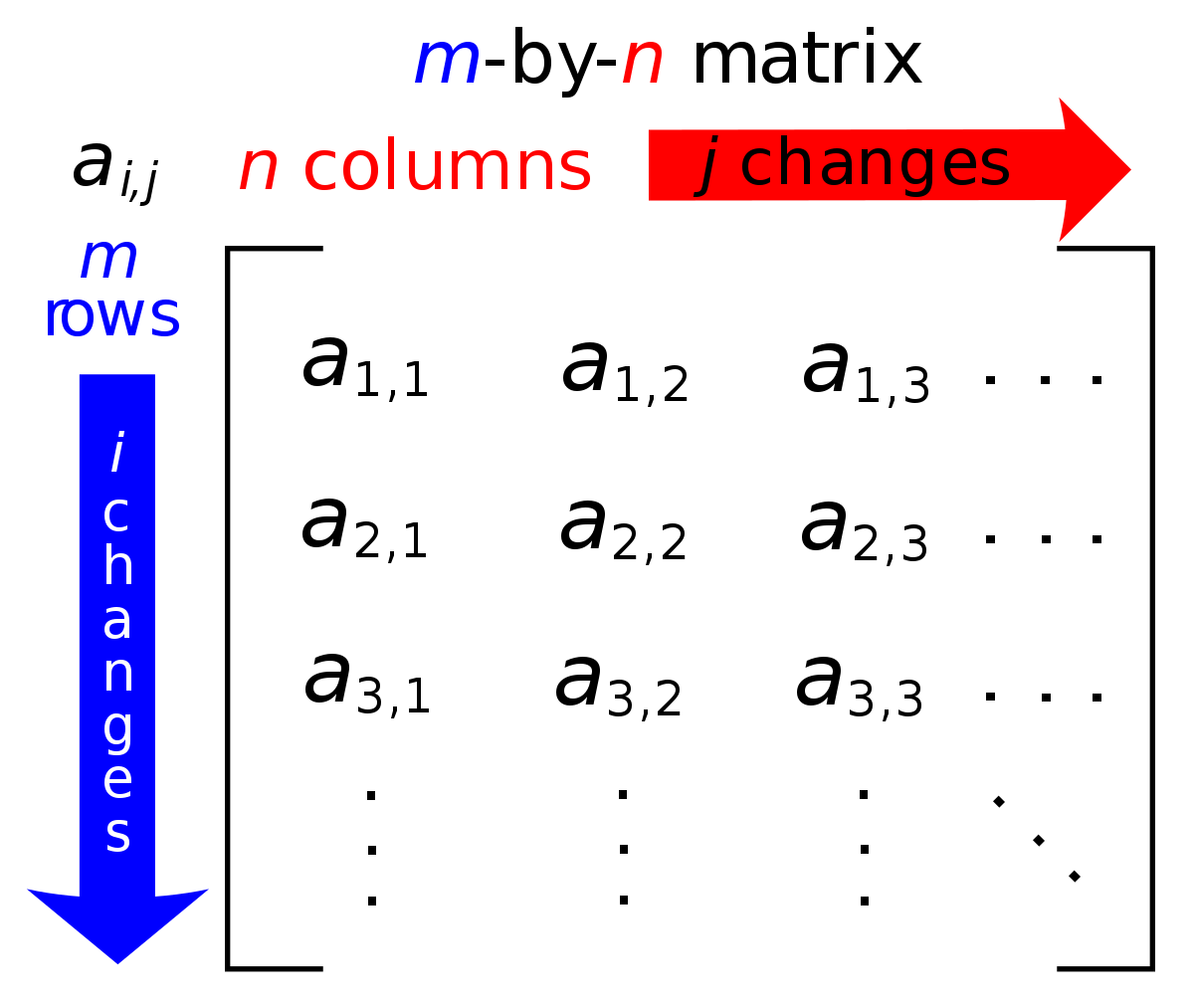color{green} ✍️ A matrix having m rows and n columns is called a matrix of order color{red}{m × n} or simply m × n matrix (read as an m by n matrix).

color{green} ✍️ In Fig the examples of matrices is shown

color{green} ✍️, we have A = [(-2,5,1),(1,2,9)] as color{green}{2× 3}

Matrix B = [(1,2,3),(4,5,6),(7,8,9)] as 3 × 3 matrix.

color{green} ✍️ Thus the i^(th) row consist of the elements a_(i1),a_(i2),a_(i3),..........,a_(in), while the j^(th) column consists of the elements a_(1j),a_(2j),a_(3j),..........,a_(mj),

color{blue}{Note :} 1. We shall follow the notation, namely A = [a_ij]_(m × n) to indicate that A is a matrix of order m × n.
2. We shall consider only those matrices whose elements are real numbers or functions taking real values.
Q 3174856756Consider the following information regarding the number of men and women workers in three factories I, II and III

Represent the above information in the form of a 3 × 2 matrix. What does the entry in the third row and second column represent?Solution:

The information is represented in the form of a 3 × 2 matrix as follows :

A = [(30,25),(25,31),(27,26)]
Q 3174056856If a matrix has 8 elements, what are the possible orders it can have?Solution:

We know that if a matrix is of order m × n, it has mn elements. Thus, to find all possible orders of a matrix with 8 elements, we will find all ordered pairs of natural numbers, whose product is 8.

Thus, all possible ordered pairs are (1, 8), (8, 1), (4, 2), (2, 4)

Hence, possible orders are 1 × 8, 8 ×1, 4 × 2, 2 × 4
Q 3174156956Construct a 3 × 2 matrix whose elements are given by a_(ij) = 1/2 |i-3j|Solution:

In general a 3 × 2 matrix is given by A= [(a_(11), a_(12)),(a_21, a_22), a_31, a_32)]

Now, a_(ij) = 1/2 |i - 3j|, i=1,2,3  and j=1,2

Therefore a_11 = 1/2 |1-3 xx 1| =1 \ a_12 =1/2 |1-3xx2| = 5/2

a_21 = 1/2 | 2- 3xx 1|=1/2 \ a_22 = 1/2 | 2-3xx2|=2

a_31 = 1/2 |3 -3 xx 1|=0 \ a_32 = 1/2 |3 -3 xx 2|=3/2

Hence the required matrix is given by A= [(1, 5/2) , (1/2 , 2), (0,3/2)]

### Types of Matrices### Column matrix

\color{green} ✍️ A matrix is said to be a column matrix if it has only one column.

\color{green} ✍️ For example, A = [(0,,),(3,,),(-1,,),(4,,)] is a column matrix of order 4 × 1.

color{green}{"In general, If n= 1 for column matrix or " A = [a_(ij)]_(m xx 1) "is a column matrix of order m × 1"}

### Row matrix

\color{green} ✍️ A matrix is said to be a row matrix if it has only one row.

Example : [ 0 \ \ -4 \ \ 3 \ \ 1]

color{green}{"In general, "B = [b_(ij)]_(1 xxn) "is a row matrix of order" 1 × n.}

### Square matrix

\color{green} ✍️ A matrix in which the number of "rows" are equal to the number of "columns", is said to be a square matrix.

Thus an m × n matrix is said to be a square matrix if m = n and is known as a square matrix of order ‘m’.

examples : [(1, -1, 0),(8,2,3),(-1,-2,6)]

color{green}{"In general, "A = [a_(ij)]_(mxx m)" is a square matrix of order m."}

### Diagonal matrix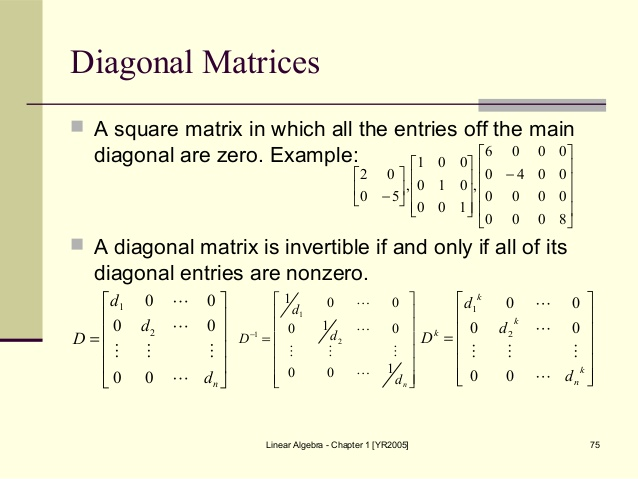color{green} ✍️ A square matrix B = [b_(ij)]_(m xx m) is said to be a diagonal matrix if color{green}{"all its non diagonal elements are zero",

color{green} ✍️ A matrix B = [b_(ij)] m xx m is said to be a diagonal matrix color{red } {if b_(ij) = 0, "when" \ \ i ≠ j

E.g. A = , B = [(-1,0),(0,-1)], c = [(-1,0,0),(0,1,0),(0,0,1)]

### Scalar matrixcolor{green} ✍️ A diagonal matrix B = [b_(ij)]_(m xx m) is said to be a scalar matrix if all its color{green}{" diagonal elements are same,"}

color{green} ✍️ A matrix B = [b_(ij)] m xx m is said to be a scalar matrix if

color{orange}{
b_(ij) = 0,\ \ "when" \ \ i ≠ j}

color{orange}{
b_(ij) = k "when" \ \ i=j}

E.g. A = , B = [(-1,0),(0,-1)], c = [(5,0,0),(0,5,0),(0,0,5)]

### Identity matrix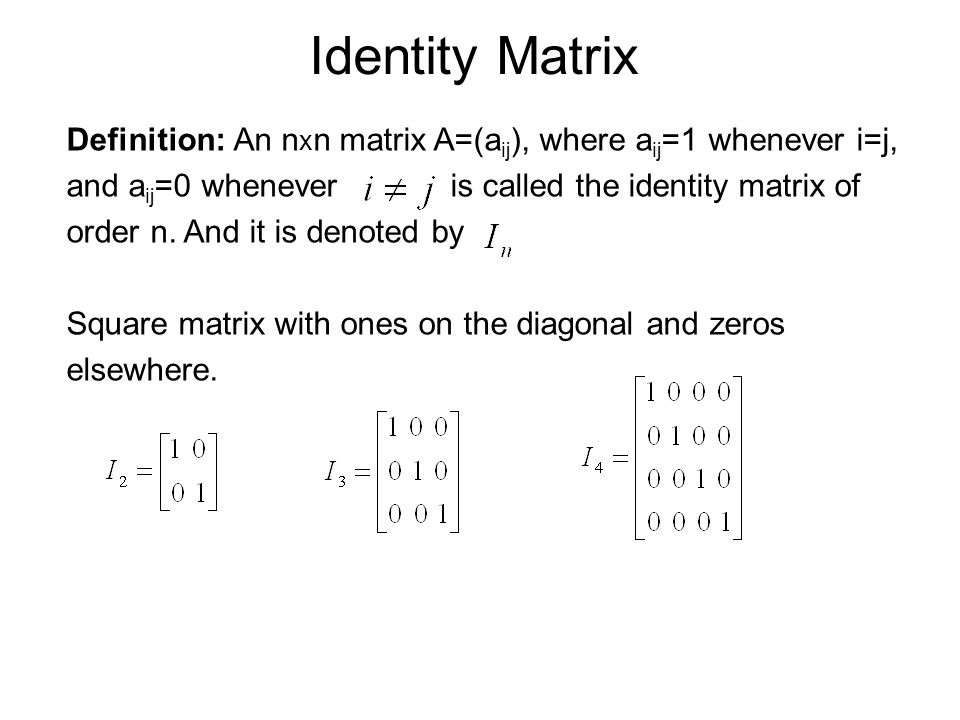color{green} ✍️ A square matrix in which elements in the diagonal are all 1 and rest are all zero is called an identity matrix. In other words, the square matrix

color{orange}{b_(ij) = 0, \ \ "when" \ \ i ≠ j}
color{orange}{b_(ij) = 1, \ \ "when" \ \ i=j}

E.g. A = , B = [(1,0),(0,1)], c = [(1,0,0),(0,1,0),(0,0,11)]

### Zero matrix

color{green} ✍️ A matrix is said to be zero matrix or null matrix if all its elements are zero.

E.g. A = , B = [(0,0),(0,0)], c = [(0,0,0),(0,0,0),(0,0,0)]

### Summery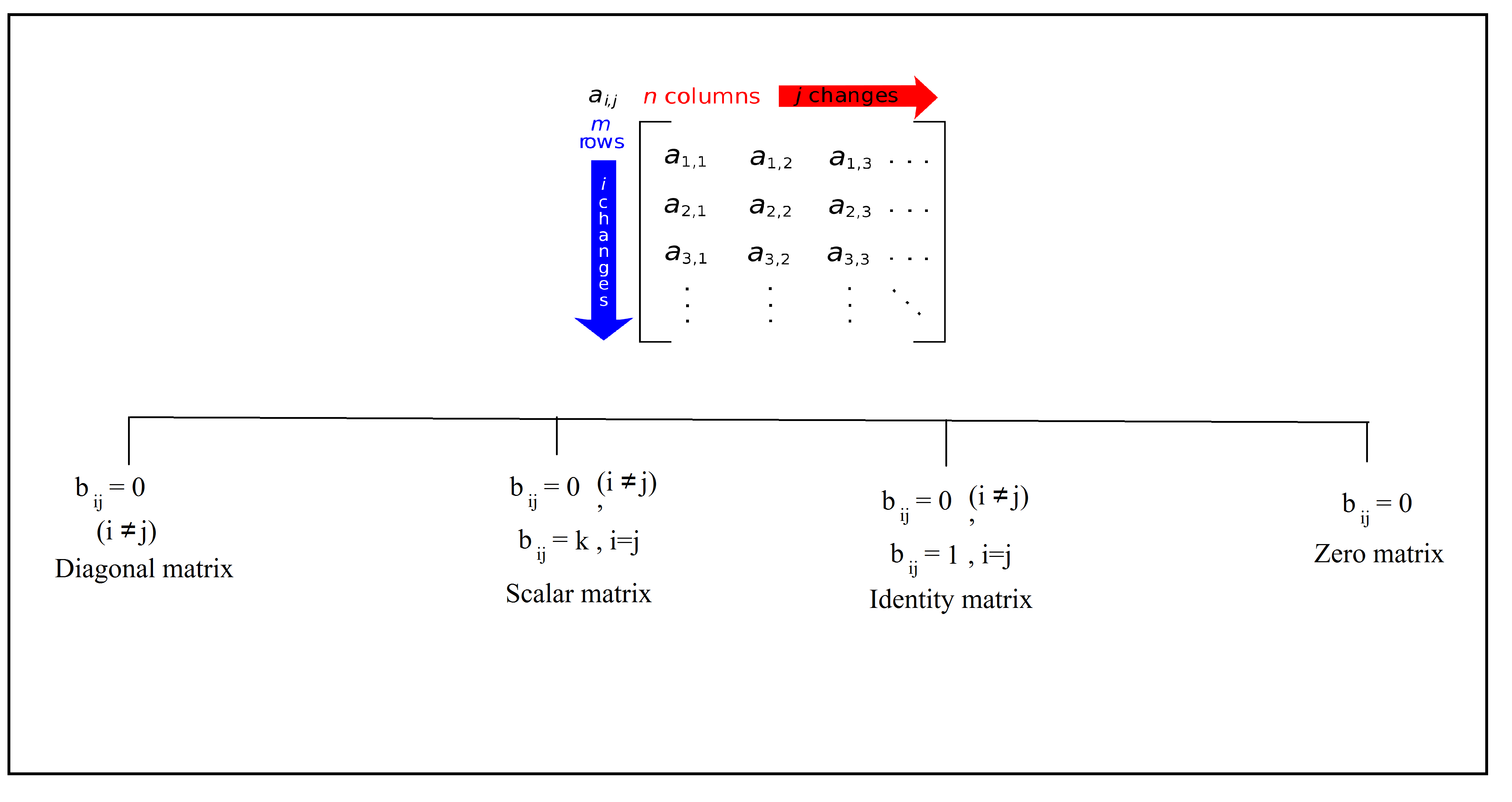### Equality of matrices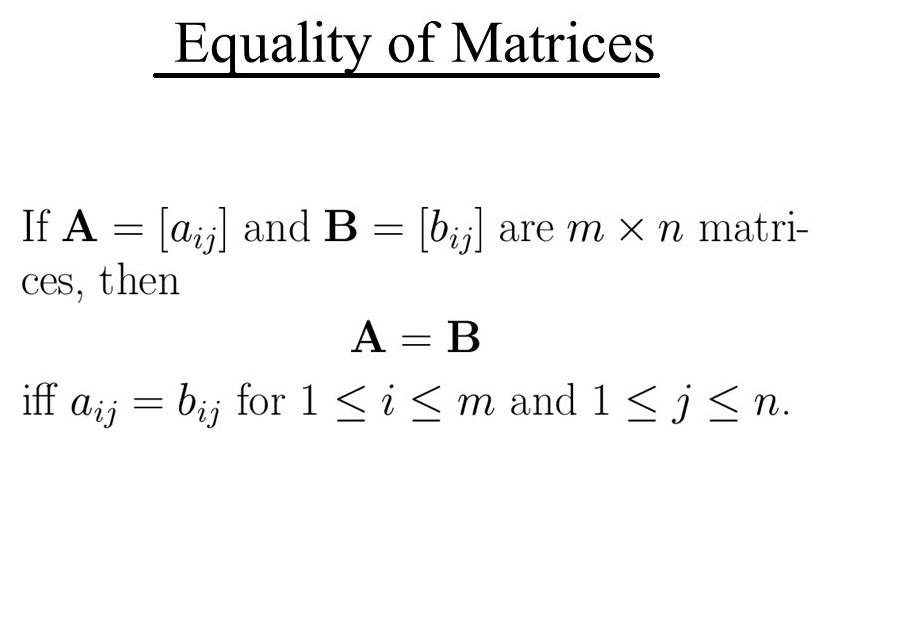\color{green} ✍️ Two matrices A = [a_(ij)] and B = [b_(ij)] are said to be equal if

(i) they are of the same order
(ii) each element of A is equal to the corresponding element of B, that is a_(ij) = b_(ij) for all i and j.

color{blue}{A = B =>[a_(ij)] = [b_(ij)]}

if [(x,y),(z,a),(b,c)] = [(-1,0),(2,sqrt6),(3,2)]  then x = -, y =0, z=2 ,a = sqrt6, b=3,c=2
Q 3124578451If  [ (x+3, z+4, 2y-7 ), (-6,a-1,0 ), ( b-3, -21 ,0 ) ] = [ (0, 6, 3y-2 ), ( -6, -3, 2c+2), ( 2b+4, -21 , 0 ) ]

Find the values of a, b, c, x, y and z.
Class 12 Chapter 3 Example 4Solution:

As the given matrices are equal, therefore, their corresponding elements
must be equal. Comparing the corresponding elements, we get

x + 3 = 0, z + 4 = 6, 2y – 7 = 3y – 2
a – 1 = – 3, 0 = 2c + 2, b – 3 = 2b + 4,

Simplifying, we get

a = – 2, b = – 7, c = – 1, x = – 3, y = –5, z = 2
Q 3144578453Find the values of a, b, c, and d from the following equation:

[ (2a+b , a-2b ), ( 5c-d, 4c +3d) ] = [ (4,-3), ( 11,24 ) ]
Class 12 Chapter 3 Example 5Solution:

By equality of two matrices, equating the corresponding elements, we get
2a + b = 4, 5c – d = 11
a – 2b = – 3 , 4c + 3d = 24

Solving these equations, we get
a = 1, b = 2, c = 3 and d = 4